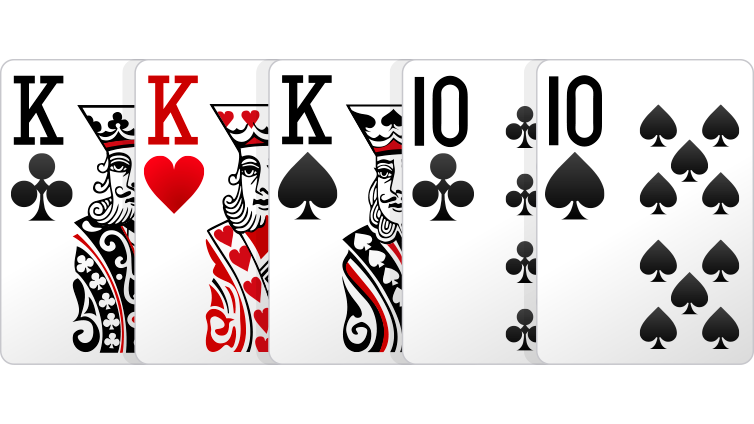# 2.4 Using Combinatorics to Calculate Probabilities

Last updated: February 20th, 2020

# Using Combinatorics to Calculate Probabilities¶

For this video, you will need to know about Combinatorics (if you don't please check here).

Imagine the following experiment: we take a hand of $5$ poker cards and we want to know the probability of getting a full house.Since all of the cards have the same probability of being drawn, this is an experiment with equally likely results.

This means that if $\Omega$ is the set of all possible combinations of $5$ cards, and we call $E$ to the set that only contains the combinations with a full house, then $P(E)=\dfrac{|E|}{|\Omega|}$.

This means that we needd to count how many elements are there in $\Omega$ and how many there are in $E$. And this is where we are going to use combinatorics.

For $|\Omega|$ we just need to find all the possible combinations of $5$ cards out of the possible $52$. Using basic combinatorics, we know that is $C(52,5)=\dfrac{52!}{(52-5)!5!}$.

In :
from math import factorial as f

In :
def C(n,r):
c = f(n)/(f(n-r)*f(r))
return int(c)

In :
C(52,5)

Out:
2598960

Now, calculating $|E|$ is a bit more complicated, but let's go through it!

For the three of a kind, we have $13$ possible numbers to choose from ($A$ to $K$). Then of that number we need to choose $3$ out of the $4$ suits, and there are $C(4,3)=4$ ways of doing that.

For the two of a kind, we now have $12$ numbers to choose from (since one of them has already been used for the three of a kind). And then of that number we need to choose $2$ out of the $4$ suits, and there are $C(4,2)=6$ ways of doing that.

So $|E|=13\times4\times12\times6$.

In :
prob = 13*4*12*6/C(52,5)
prob

Out:
0.0014405762304921968